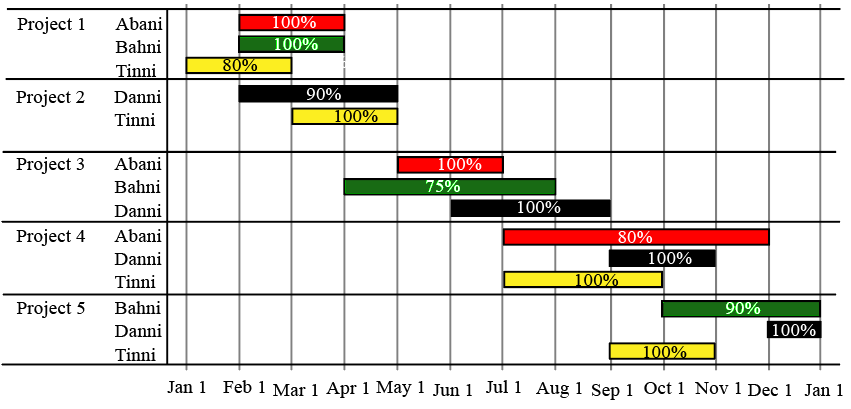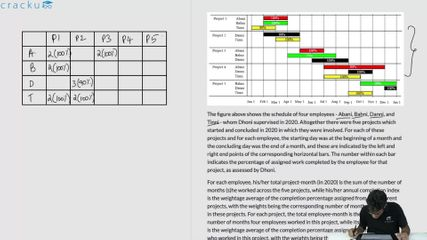### CAT 2021 Question Paper (Slot 3) Question 29

InstructionsThe figure above shows the schedule of four employees - Abani, Bahni, Danni, and Tinni - whom Dhoni supervised in 2020. Altogether there were five projects which started and concluded in 2020 in which they were involved. For each of these projects and for each employee, the starting day was at the beginning of a month and the concluding day was the end of a month, and these are indicated by the left and right end points of the corresponding horizontal bars. The number within each bar indicates the percentage of assigned work completed by the employee for that project, as assessed by Dhoni.

For each employee, his/her total project-month (in 2020) is the sum of the number of months (s)he worked across the five projects, while his/her annual completion index is the weightage average of the completion percentage assigned from the different projects, with the weights being the corresponding number of months (s)he worked in these projects. For each project, the total employee-month is the sum of the number of months four employees worked in this project, while its completion index is the weightage average of the completion percentage assigned for the employees who worked in this project, with the weights being the corresponding number of months they worked in this project.

Question 29

# Which of the following statements is/are true?I: The total project-month was the same for the four employees.II: The total employee-month was the same for the five projects.

Solution

The total project month is the number of months Abani, Bahni, Danni, and Tinni individually worked for all the projects combined :

Abani - 2+2+5 = 9 months

Bahni - 2+4+3 = 9 months

Danni - 3+3+2+1 = 9 months

Tinni - 2+2+3+2 = 9 months.

The total employee month for all the five projects is the sum of the total employee-month is the sum of the number of months four employees worked in this project.

Project -1 = 2+2+2 = 6 months

Project -2 = 3+2 = 5 months

Project - 3 = 2+4+3 = 9 months.

Project - 4 = 5+2+3 = 10 months.

Project - 5 = 3+1+2 = 6 months.

Only statement 1 is true.

### View Video Solution• All Quant CAT Formulas and shortcuts PDF
• 30+ CAT previous papers with solutions PDF

##### Harsha Agarwal

1 year, 3 months ago

Hi sir, i bought cracku's crash course today and would really love to opt for refund seeing that whatever i was expecting hasn't been provided. Also i tried emailing, reaching out to support staff, but no good redressal has been given. Pls help me the same?? :(

##### Gayatri Dalu

1 year ago

you are right....I also tried reaching to staff bt we cant directly contact with them its too sad.and also i m not getting what sayali mam teach they dont even elaborate the steps of ans and where that ans is coming from.

##### Harsha Agarwal

1 year, 3 months ago

On call i made it clear what i was expecting, and the representative told me that its there but it wasn't. It was really sad